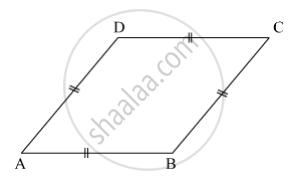SSC (English Medium) Class 8Maharashtra State Board
Share

# Measure of One Angle of a Rhombus is 50° .Find the Measures of Remaining Three Angles. - SSC (English Medium) Class 8 - Mathematics

ConceptSome Special Parallelograms Concept of Rhombus

#### Question

Measure of one angle of a rhombus is 50° .find the measures of remaining three angles.

#### Solution

Suppose ABCD is a rhombus.Let m∠A = 50º.

Opposite angles of a rhombus are congruent.

∴ m∠C = m∠A = 50º and m∠B = m∠D

Now,

m∠A + m∠B  + m∠C + m∠D = 360º

∴ 50º + m∠B  + 50º + m∠D = 360º

⇒ m∠B + m∠D = 360º − 100º = 260º

⇒ 2 m∠B = 260º               (m∠B = m∠D)

⇒ m∠B = (260°)/2 = 130°

∴ m∠D = m∠B = 130º

Thus, the measures of the remaining angles of the rhombus are 130º, 50º and 130º.

Is there an error in this question or solution?

#### APPEARS IN

Balbharati Solution for Balbharati Class 8 Mathematics (2019 to Current)
Chapter 8: Quadrilateral : Constructions and Types
Practice Set 8.2 | Q: 7 | Page no. 47
Solution Measure of One Angle of a Rhombus is 50° .Find the Measures of Remaining Three Angles. Concept: Some Special Parallelograms - Concept of Rhombus.
S